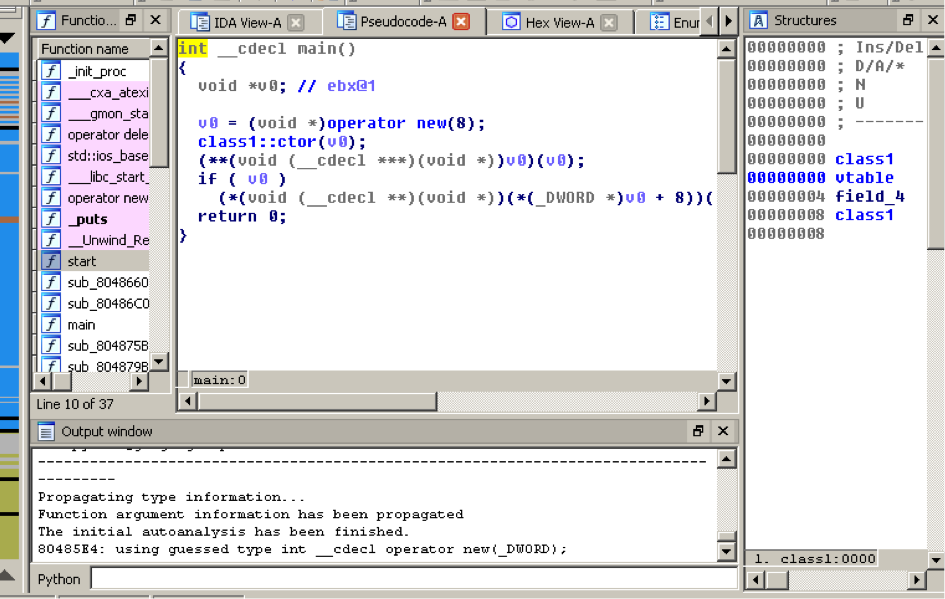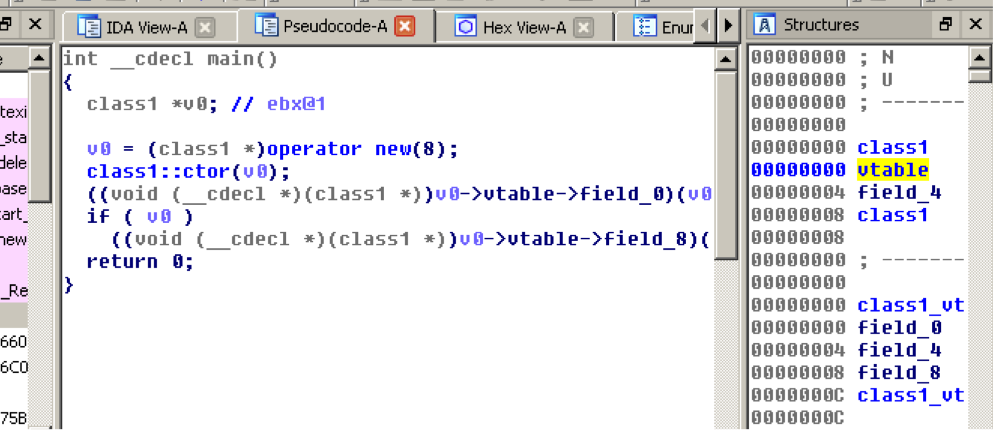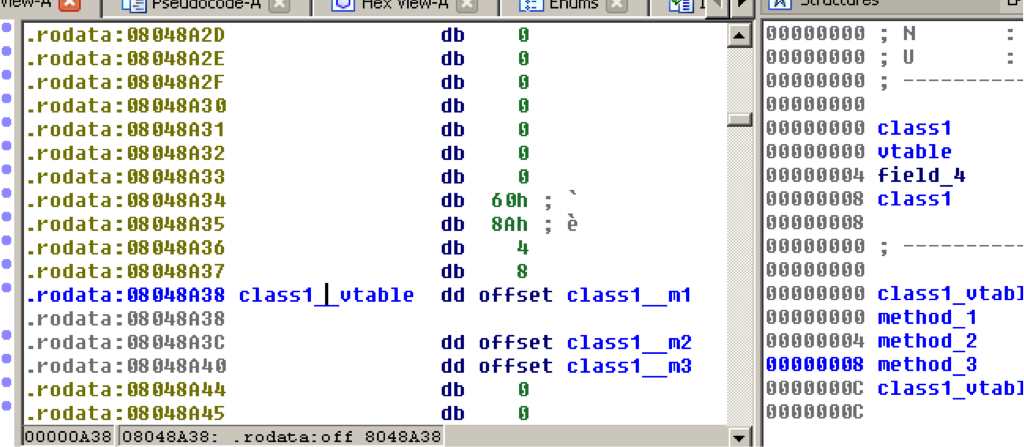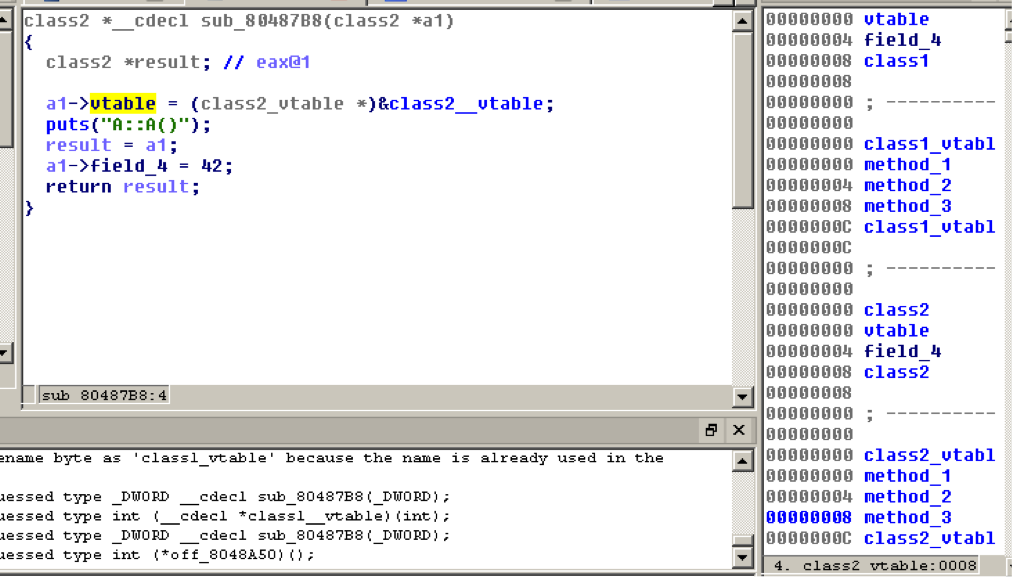# 简介

v81 = 9;
v63 = *(_DWORD *)(v62 + 88);
if ( v63 )
{
v64 = *(int (__cdecl **)(_DWORD, _DWORD, _DWORD,
_DWORD, _DWORD))(v63 + 24);
if ( v64 )
v62 = v64(v62, v1, *(_DWORD *)(v3 + 16), *(_DWORD
*)(v3 + 40), bstrString);
}


padding = *Dst;
if ( padding < 4 )
return -1;
buffer_skip_bytes(this2->decrypted_input_buffer, 5u);
if ( this2->encrypt_in != null )
{
if ( this2->compression_in != null )
{
buffer_reinit(this2->compression_buffer_in);
packet_decompress(this2,
this2->decrypted_input_buffer,
this2->compression_buffer_in);
buffer_reinit(this2->decrypted_input_buffer);
avail_len = buffer_avail_bytes(this2->compression_buffer_in);
ptr = buffer_get_data_ptr(this2->compression_buffer_in);
}
}
packet_type = buffer_get_u8(this2->decrypted_input_buffer);
*len = buffer_avail_bytes(this2->decrypted_input_buffer);
this2->packet_len = 0;
return packet_type;


# C++程序的结构

Class = data structure + code (methods).


Object = memory allocation + data + virtual functions.


## 对象的创建

int __cdecl sub_80486E4()
{
void *v0; // ebx@1
v0 = (void *)operator new(8);
sub_8048846(v0);
(**(void (__cdecl ***)(void *))v0)(v0);
if ( v0 )
(*(void (__cdecl **)(void *))(*(_DWORD *)v0 + 8))(v0);
return 0;
}


## 重命名符号名称

int __cdecl main()
{
void *v0; // ebx@1
v0 = (void *)operator new(8);
class1::ctor(v0);
(**(void (__cdecl ***)(void *))v0)(v0);
if ( v0 )
(*(void (__cdecl **)(void *))(*(_DWORD *)v0 + 8))(v0);
return 0;
}


## 创建结构

IDA的结构（structures）窗口非常有用。按shitf + f9能够调出来。我建议你把它拖出来放到IDA窗口的右边（IDA的QT版能这么做），然后你就能同时看到反汇编窗口和结构窗口。## 分析构造函数

int __cdecl class1::ctor(void *a1)
{
sub_80487B8(a1);
*(_DWORD *)a1 = &off_8048A38;
return puts("B::B()");
}


sub_80487B8() 在构造函数里被直接调用，这个函数也许是class1的经函数，但也可能是父类的构造函数。

off_8048A38是class1的vtable，到这里你已经能知道vtable的大小了（只需要看vtable附近有Xref的数据的数量）和一个class1虚函数的列表。你可以把他们命名成“ class1_mXX”，但需要注意的是其中的一些函数可能与其他类共享。int __cdecl sub_80487B8(int a1)
{
int result; // eax@1
*(_DWORD *)a1 = &off_8048A50;
puts("A::A()");
result = a1;
*(_DWORD *)(a1 + 4) = 42;
return result;
}


1. 看对class2 ::ctor的xref，如果我们能找到一个对它的直接调用，例如一个对class2的实例化，我们就能知道class2成员函数的大小。
2. 看vtable里的函数，尝试找出被访问过的最高的成员。## 对析构函数的研究

Let’s go back to our main function. We can see that the last call, before our v0 object becomes a memory leak, is a call to the third virtual method of class2. Let’s study it.

if ( v0 )
((void (__cdecl *)(class1 *))
v0->vtable->method_3)(v0);

void __cdecl class1::m3(class1 *a1)
{
class1::m2(a1);
operator delete(a1);
}

void __cdecl class1::m2(class1 *a1)
{
a1->vtable = (class1_vtable *)&class1__vtable;
puts("B::~B()");
class2::m2((class2 *)a1);
}

void __cdecl class2::m2(class2 *a1)
{
a1->vtable = (class2_vtable *)&class2__vtable;
puts("A::~A()");
}


## 这些映射是怎么回事，为什么两个结构里定义了一样的变量？

classXX 包含
+++ vtable (typed to classXX_vtable *)
+++ classXX-1_members (members of the superclass)
+++ classXX_members, if any
classXX_vtable contains
+++classXX-1_vtable
+++classXX’s vptrs, if any

00000000 class1          struc ; (sizeof=0x8)
00000000 vtable          dd ?                    ; offset
00000004 class2_members  class2_members ?
00000008 class1          ends
00000008
00000000 ; ----------------------------------------------00000000
00000000 class1_members  struc ; (sizeof=0x0)
00000000 class1_members  ends
00000000
00000000 ; ----------------------------------------------00000000
00000000 class1_vtable   struc ; (sizeof=0xC)
00000000 class2_vtable   class2_vtable ?
0000000C class1_vtable   ends
0000000C
00000000 ; ----------------------------------------------00000000
00000000 class2          struc ; (sizeof=0x8)
00000000 vtable          dd ?                    ; offset
00000004 members         class2_members ?
00000008 class2          ends
00000008
00000000 ; ----------------------------------------------00000000
00000000 class2_vtable   struc ; (sizeof=0xC)
00000000 method_1        dd ?                    ; offset
00000004 dtor            dd ?                    ; offset
00000008 delete          dd ?                    ; offset
0000000C class2_vtable   ends
0000000C
00000000 ; ----------------------------------------------00000000
00000000 class2_members  struc ; (sizeof=0x4)
00000000 field_0         dd ?
00000004 class2_members  ends
00000004

int __cdecl main()
{
class1 *v0; // ebx@1
v0 = (class1 *)operator new(8);
class1::ctor(v0);
((void (__cdecl *)(class1 *)) v0->vtable->class2_vtable.method_1)(v0);
if ( v0 )
((void (__cdecl *)(class1 *)) v0->vtable->class2_vtable.delete)(v0);
return 0;
}

int __cdecl class1::ctor(class1 *a1)
{
class2::ctor((class2 *)a1);
a1->vtable = (class1_vtable *)&class1__vtable;
return puts("B::B()");
}

class2 *__cdecl class2::ctor(class2 *a1)
{
class2 *result; // eax@1
a1->vtable = (class2_vtable *)&class2__vtable;
puts("A::A()");
result = a1;
a1->members.field_0 = 42;
return result;
}


# 总结

1. 当你找到一个新的类时，对其进行命名，在分析出这个类的有意义的名字前分析出整个继承树。
2. 从父类开始分析到子类。
3. 先查看构造函数和析构函数，找到对new()和静态方法的调用。
4. 同一个类的函数在编译过的文件里一般彼此相邻。而相关的类（继承关系）可能彼此之间离得很远。有时候构造函数会在子类的构造函数里内联，甚至在实例化的地方出现。
5. 如果你想在逆向继承关系比较复杂的结构时，使用“结构包含结构”的技巧只需要命名一次变量。
6. 尽管使用hex-rays的类型系统，它非常强大。
7. 纯虚类很让人头大，你可以发现几个类有相似的vtable，但却通常没有代码，要注意他们。

# 本文中用到的代码

#include <iostream>
#include <stdio.h>

class A {
public:
A(){
printf("A::A()\n");
id = 42;
}
virtual void a(){
printf("Virtual A::a()\n");
}
virtual ~A(){
printf("A::~A()\n");
}
private:
int id;
};

class B : public A {
public:
B(){
printf("B::B()\n");
}
virtual ~B(){
printf("B::~B()\n");
}
virtual void a(){
printf("Virtual B::a()\n");
A::a();
}
};

int main(){
A *b = new(B);
b->a();
delete(b);
return 0;
}


posted @ 2018-12-25 13:44  Antiver  阅读(16266)  评论(0编辑  收藏